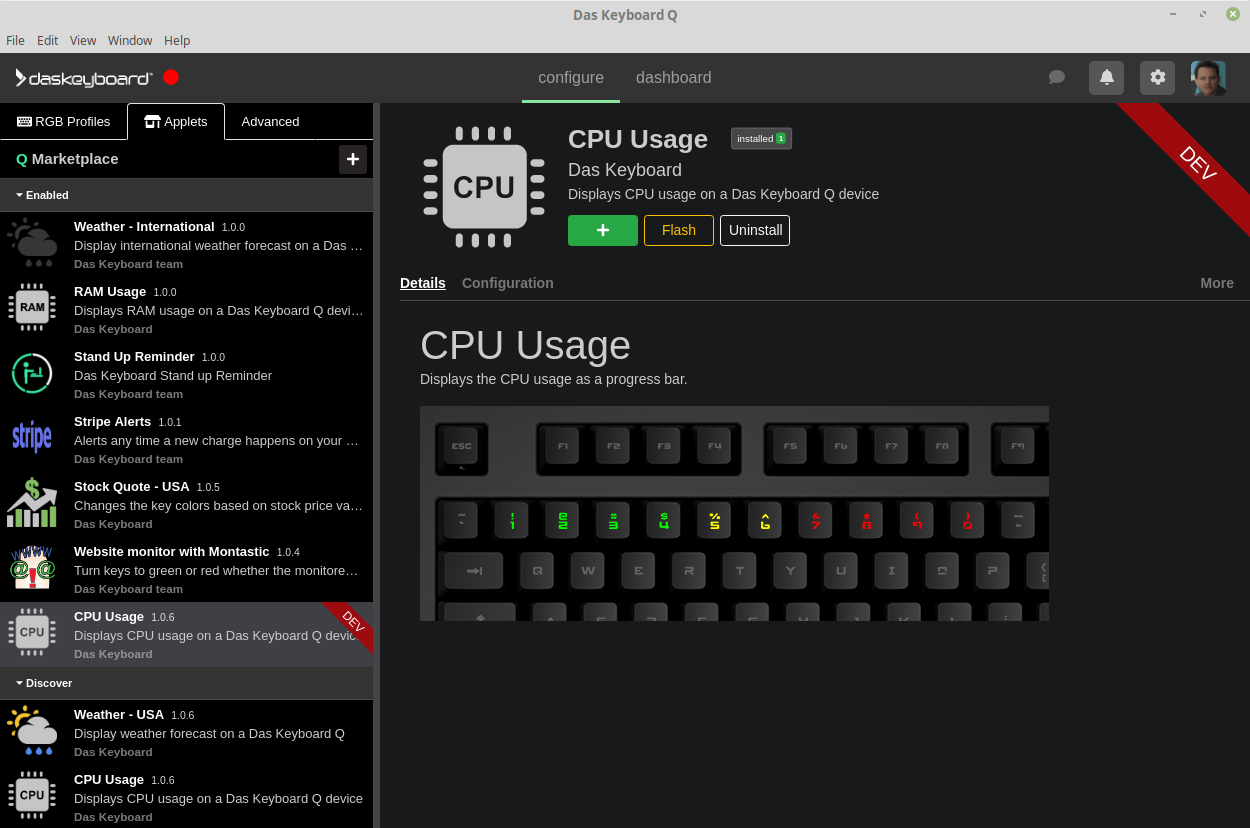Howdy developers. You’ll find here the information needed to develop Q applets for Das Keyboard Q devices.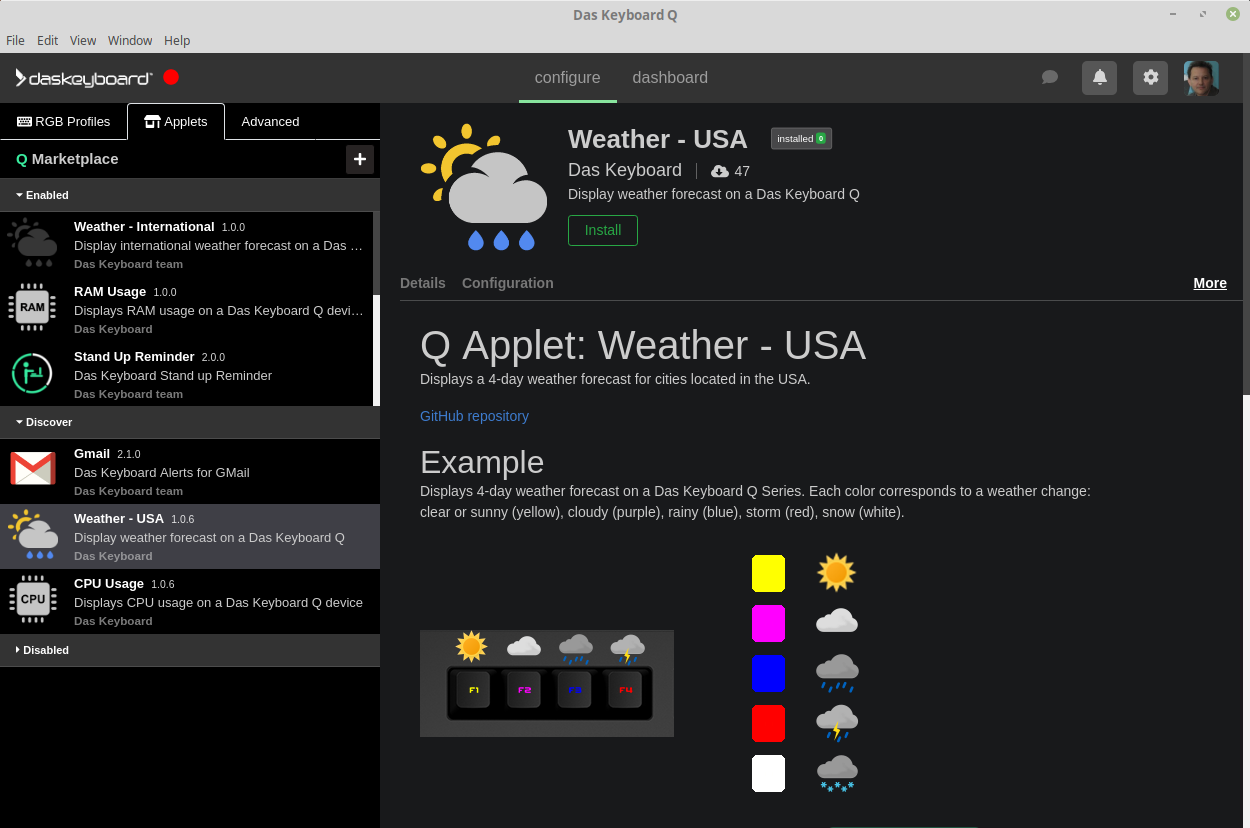Q applet development is easy. There are many examples available, all open source.

A Q applet is a program written in `javascript` that can be installed in the Q software. Applets live in the Q marketplace.

Applets control a user-defined set of RGB LEDs. For example, a `CPU meter` would control the top horizontal LEDs from `backtick` to `9` (US layout).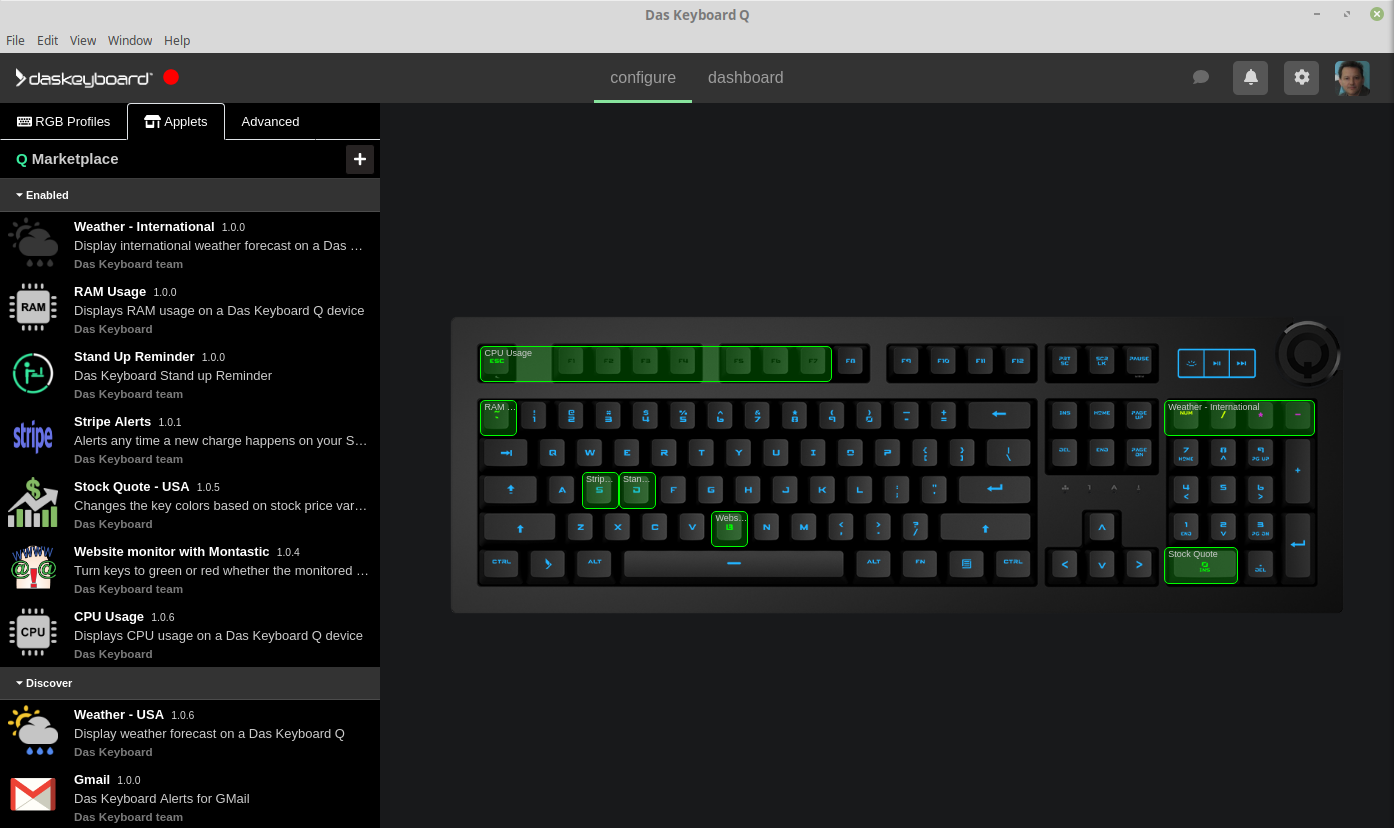Here is the source code of full-featured applets. Clone as needed.

• Cpu monitor applet:
• Weather forecast applet
• Stock quote applet:
• Many more Q applets:

## Key concepts

A Q applet controls LEDs inside a `rectangular area` which is a 2D array of points.

Example 1: a `CPU activity meter` could use 10 LEDs on one row.

``````return new q.Signal({
points: [[
new q.Point('#FF0000),
new q.Point('#00FF00),
//.... 10 points total
new q.Point('#0000FF),
]],
name: 'CPU 28%',
});
``````

Example 2: a `stock applet` of one stock ticker would display itsself on one LED only. A user can add many stock applet instances.

``````return new q.Signal({
points: [[
new q.Point('#0000FF)
]],
name: 'TSLA up 25%',
description: 'Tesla: \$1780'
});
``````

## Applet development overview: making a CPU meter

### Prerequisites

Make sure `node` and `yarn` are installed.

Let’s walk through a concrete example and write a CPU meter applet that displays a bargraph on a Q keyboard. The final example is available on Github: https://github.com/daskeyboard/daskeyboard-applet--cpu-monitor. Clone it to follow along.

``````git clone https://github.com/daskeyboard/daskeyboard-applet--cpu-usage.git
``````

then install `node modules`:

``````yarn
``````

### File structure

A typical Q applet file structure will look like this:

``````> tree
.
├── assets
│   ├── icon.png
│   └── image.png
├── CHANGELOG.md
├── package.json
├── index.js
└── yarn.lock
``````

The app packages dependencies are listed in `package.json` file.

The `assets` directory contains the images for the marketplace.

The `README_ENDUSER.md` is the content that will be shown in the marketplace.

### Defining which LEDs to use: this.geometry

The Q applet will define its rectangle area needs in the `package.json` file as follows. In the example below, the applet uses 10 LEDs (`width`) over 1 keyboard row (`height`). During the installation by the end user, the applet will be first positioned at `orign` point (0,1) here which correspond to the `ESCAPE` key. The end user will then drag and drop the applet to a location of his or her choice.

The `height`, `width` and `origin` are defined by the `qConfig` JSON structure in package.json with the format:

``````{
"width": <number>
"height": <number>
"origin": {
"x": <number>,
"y": <number>
}
}
``````

You can also inspect the applet’s geometry with the functions:

• `this.getWidth()`
• `this.getHeight()`
• `this.getOriginX()`
• `this.getOriginY()`
`````` "qConfig": {
"geometry": {
"width": 10,
"height": 1,
"defaults": {
"origin": {
"x": 0,
"y": 1
}
}
``````

The above `qConfig` settings will display the applet (orange rectangle) as follows: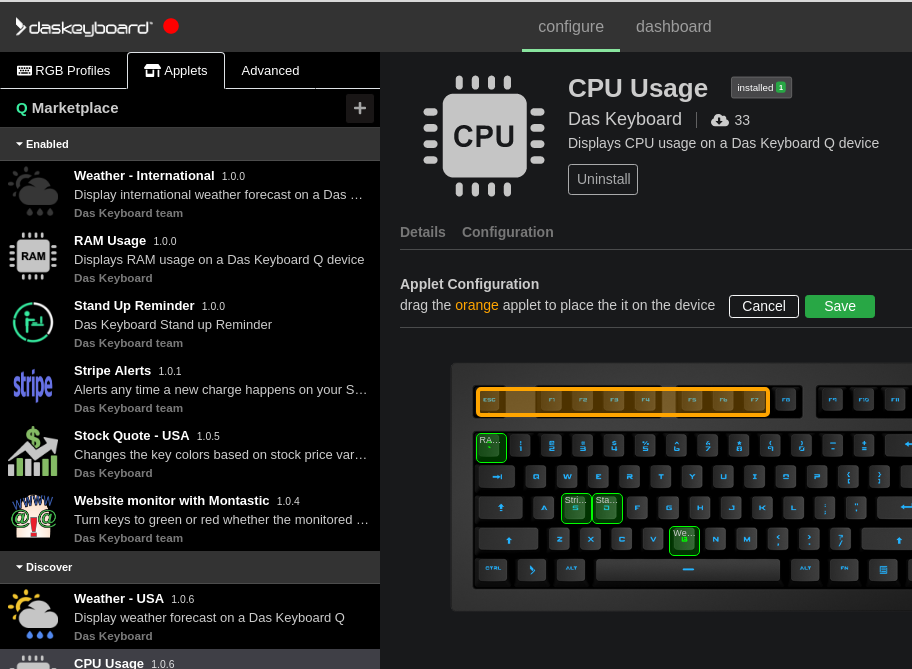Applet’s geometry can be inspected with the functions:

``````this.getWidth();
this.getHeight();
this.getOriginX();
this.getOriginY();
``````

### Polling interval

The heart of the Q applet is the `javascript` file `index.js`. Let’s dive into it.

In the case of the CPU meter, we would like to update the RGB LEDs of the keyboard every 3 seconds.

To achieve this, we set the `polling interval` to 3000 milliseconds `this.pollingInterval = 3000;` in the applet class contructor as follows:

``````class CpuUsage extends q.DesktopApp {
constructor() {
super();
// run every 3000 ms
this.pollingInterval = 3000;
logger.info("CPU Usage Meter ready to go!");
}
``````

Then we write the `run` function that will be called each `pollingInterval`. The CPU meter will send a signal to the keyboard each time the `run` function is called.

`getCpuUsage` returns the percentage of CPU usage then the LED color and how many LEDs to use is calculated with `generatePoints(percent)`:

`````` // call this function every pollingInterval
// sends a signal to the Q keyboard to light up LEDs based on CPU usage.
async run() {
return this.getCpuUsage().then(percent => {
return new q.Signal({
points: [this.generatePoints(percent)],
name: "CPU Usage",
message: Math.round(percent * 100) + "%",
isMuted: true, // don't flash the Q button on each signal
});
});
}
``````

Here `this.getCpuUsage()` calculates the CPU usage percentage:

``````  async getCpuUsage() {
return new Promise((resolve) => {
os.cpuUsage(v => { // ask value from OS.
resolve(v);
})
})
}
``````

This is how `generatePoints` calculates how many LEDs should be used:

`````` generatePoints(percent) {
const numberOfKeys = 10;
// multiply the cpu percentage by the number total of keys
const numberOfKeysToLight = Math.round(numberOfKeys * percent);
let points = [];

// create a list of points (zones) with a color). Each point
// correspond to an LED
for (let i = 0; i < numberOfKeys; i++) {
points.push(new q.Point(this.getColor(i, numberOfKeysToLight)));
}

return points;
}
``````

Finally, here is the complete content of `index.js` file:

``````// Library to track cpuUsage
const os = require('os-utils');

// Library to send signal to Q keyboards

// Color associated to cpu activity from low (green) to high (red).
const colors = ['#00FF00', '#00FF00', '#00FF00', '#00FF00', '#FFFF00', '#FFFF00', '#FF0000',
'#FF0000', '#FF0000', '#FF0000'
];

const logger = q.logger;

class CpuUsage extends q.DesktopApp {
constructor() {
super();
// run every 3000 ms
this.pollingInterval = 3000;
logger.info("CPU Usage Meter ready to go!");
}

// call this function every pollingInterval
async run() {
return this.getCpuUsage().then(percent => {
return new q.Signal({
points: [this.generatePoints(percent)],
name: "CPU Usage",
message: Math.round(percent * 100) + "%",
isMuted: true, // don't flash the Q button on each signal
});
});
}

async getCpuUsage() {
return new Promise((resolve) => {
os.cpuUsage(v => {
resolve(v);
})
})
}

generatePoints(percent) {
const numberOfKeys = 10;
// multiply the cpu percentage by the number total of keys
const numberOfKeysToLight = Math.round(numberOfKeys * percent);
let points = [];

// create a list of points (zones) with a color). Each point
// correspond to an LED
for (let i = 0; i < numberOfKeys; i++) {
points.push(new q.Point(this.getColor(i, numberOfKeysToLight)));
}

return points;
}

/** get a color of a zone depending on it's index on the zone array */
getColor(zoneIndex, numberOfKeysToLight) {
if (zoneIndex >= numberOfKeysToLight) {
// if the zone is after the number max of keys to light. Turn off the light
// Black color = no light
return '#000000';
} else {
// turn on the zone with the proper color
return colors[zoneIndex];
}
}
}

module.exports = {
CpuUsage: CpuUsage
};

const cpuUsage = new CpuUsage();
``````

A during development, an applet can be loaded into Das Keyboard Q desktop.

This allows to check the applet assets such as images, READMEs, config view and overall applet behavior and user experience.

Before loading the applet inside Q desktop, the `node modules` need to be installed using the following command:

``````yarn
``````

The applet can be loaded via the `dev menu` `</>`. A user can enable from the user setting menu.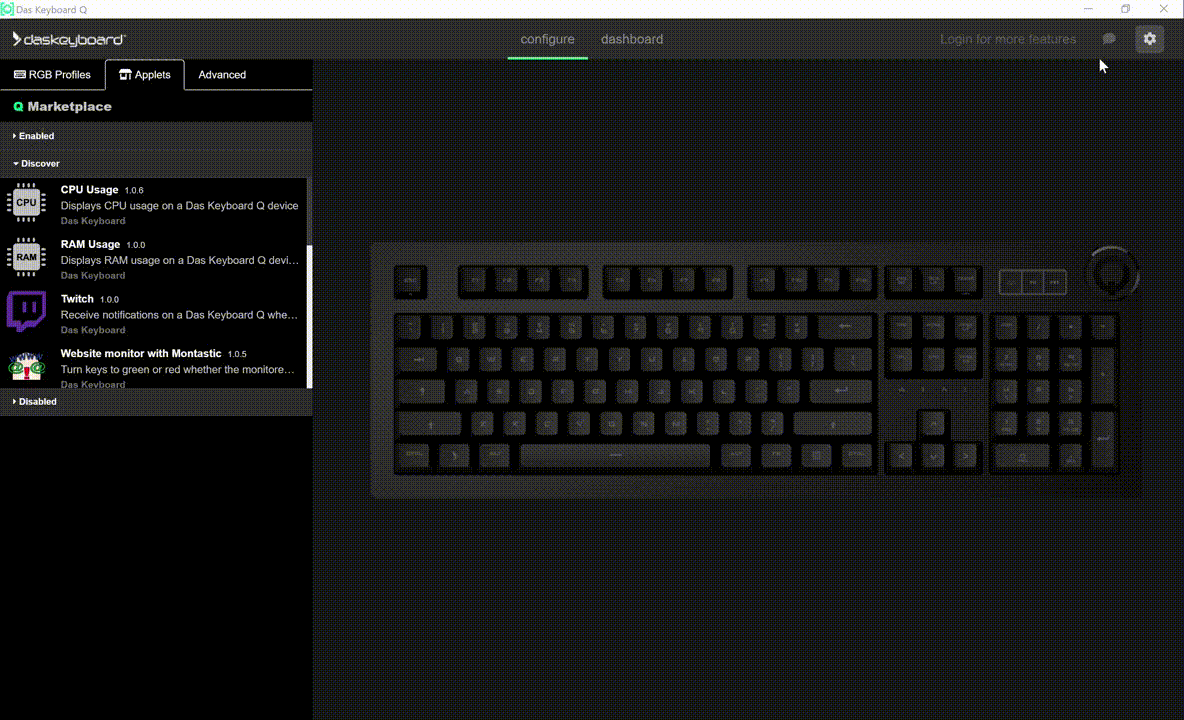Here is a view of the `CPU Usage` applet loaded in dev mode inside Q desktop application.